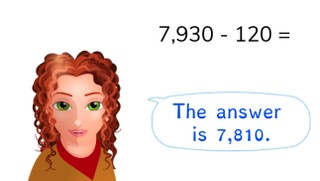Subtraction to 10,000 without regrouping

# Subtraction to 10,000 without regrouping

Students learn to subtract to 10,000 without regrouping.

No account needed.8,000 schools use Gynzy92,000 teachers use Gynzy1,600,000 students use Gynzy

## General

The students learn to subtract to 10,000 without regrouping.

## Standards

CCSS.Math.Content.4.NBT.B.4

## Learning objective

Students will be able to subtract to 10,000 without regrouping.

## Introduction

Have the students solve the problems together.

## Instruction

Explain that each cube always contains 1,000 blocks, each sheet 100 blocks and each row 10 blocks. That is how you can quickly count how many blocks you see in the image. Then show the problem 7,930 - 120. Count the blocks on the page together. Count how many you have in total, and then how many are taken away and how many blocks you have left. Discuss solving the problem 3,997 - 564 = in the same way. Afterwards, have the students solve some problems on their own. Then show the number line and explain that you can solve the problem 5,758 - 631 by first subtracting the hundreds numbers, then the tens numbers and finally the ones values. Next, discuss the different ways to solve 3,890 - 670 and have the students solve the next few problems on their own. Finally, discuss the steps to solve a story problem. First determine what type of problem it is and what numbers you need, then state the problem and solve it. Discuss the problem about the popsicles together and have the students solve the next problem on their own.

Check whether the students can subtract to 10,000 without regrouping by asking the following question:
- Which method do you use to solve this problem in steps? (2,230 - 130 = ...)

## Quiz

The students are given ten problems in which they practice with visual, abstract, and story problems.

## Closing

Discuss again the importance of being able to subtract to 10,000 without regrouping. As a closing activity, have the students solve the story problem in pairs.

## Teaching tips

Have students have have difficulty with subtracting to 10,000 without regrouping first practice subtracting to 10,000 with simple numbers.

### The online teaching platform for interactive whiteboards and displays in schools

• Save time building lessons

• Manage the classroom more efficiently

• Increase student engagement